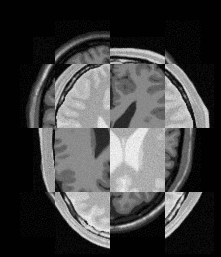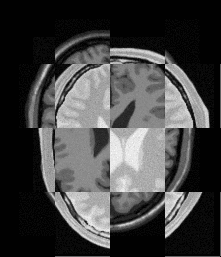How to tune registration

aThe result seems very unsatisfactory，and my weight adjustment has no effect on the result

You might need to increase optimizer’s initial step size, allow for more iterations, have a better initialization, etc. Achieving that with a more complicated setup (multi-metric) is harder than with a simpler setup (e.g. mutual information metric). Try to make it work with a simpler setup before making it more complicated. To presumably make it work for larger variety of cases?

1 Like

That looks like the initial location is poor and a large distance needs to be traveled by the registration optimization.

Consider removed the high frequencies with smoothing and using a multi-scale/resolution approach.

I feel I’m a little off track. What I need to do is 2D-3D multimodal registration of blood vessels. I wanted to find a good metric for 2D-3D registration from 2D-2D multimodal registration.The effect of 2d-3d registration is very bad. My code is as follows:

int main(int argc, char* argv[])
{
double projAngle1 =0.;
// Default output file names
const char* fileOutput1 = "out_put.tif";

bool ok;
bool verbose = true;
bool debug = false;
bool customized_iso = false;
bool customized_2DCX = false;   // Flag for customized 2D image central axis positions
bool customized_2DRES = true; // Flag for customized 2D image pixel spacing

double rx = 0.;
double ry = 0.;
double rz = 0.;

double tx = -5.;
double ty = 0.;
double tz = -5.;

double cx = 0.;
double cy = 0.;
double cz = 0.;

double scd = 1061.; // Source to isocenter distance

double image1centerX = 0.0;
double image1centerY = 0.0;

double image1resX = 0.37;
double image1resY = 0.37;

double threshold = 0.0;

constexpr unsigned int Dimension = 3;
using InternalPixelType = float;
using PixelType3D = short;

using ImageType3D = itk::Image<PixelType3D, Dimension>;

using OutputPixelType = unsigned char;
using OutputImageType = itk::Image<OutputPixelType, Dimension>;

// The following lines define each of the components used in the
// registration: The transform, optimizer, metric, interpolator and
// the registration method itself.

using InternalImageType = itk::Image<InternalPixelType, Dimension>;

using TransformType = itk::Euler3DTransform<double>;
/*using TransformType = itk::Rigid3DTransform<double>;*/

using OptimizerType = itk::PowellOptimizer;

/*using MetricType = itk::NormalizedCorrelationImageToImageMetric<InternalImageType, InternalImageType>;*/
using MetricType = itk::MutualInformationImageToImageMetric<InternalImageType, InternalImageType>;
using InterpolatorType = itk::SiddonJacobsRayCastInterpolateImageFunction<InternalImageType, double>;
using RegistrationType = itk::ImageRegistrationMethod<InternalImageType, InternalImageType>;

MetricType::Pointer       metric = MetricType::New();
TransformType::Pointer    transform = TransformType::New();
OptimizerType::Pointer    optimizer = OptimizerType::New();
InterpolatorType::Pointer interpolator1 = InterpolatorType::New();
RegistrationType::Pointer registration = RegistrationType::New();

/* metric->SetSubtractMean(false);*/

registration->SetMetric(metric);
registration->SetOptimizer(optimizer);
registration->SetTransform(transform);
registration->SetInterpolator(interpolator1);

metric->SetFixedImageStandardDeviation(0.4);
metric->SetMovingImageStandardDeviation(0.4);

using ImageIOTypeNII = itk::NiftiImageIO;
ImageIOTypeNII::Pointer NiiImageIO = ImageIOTypeNII::New();

// To simply Siddon-Jacob's fast ray-tracing algorithm, we force the origin of the CT image
// to be (0,0,0). Because we align the CT isocenter with the central axis, the projection
// geometry is fully defined. The origin of the CT image becomes irrelavent.
//为了简化Siddon Jacob的快速光线跟踪算法，我们强制CT图像的原点为（0,0,0）。因为我们将CT等中心与中心轴对齐，
//所以投影几何体已完全定义。CT图像的来源变得无关
ImageType3D::PointType image3DOrigin;
image3DOrigin = 0.0;
image3DOrigin = 0.0;
image3DOrigin = 0.0;
image3DIn->SetOrigin(image3DOrigin);

if (customized_2DRES)
{
InternalImageType::SpacingType spacing;
spacing = image1resX;
spacing = image1resY;
spacing = 1.0;
image_tmp1->SetSpacing(spacing);
}

// The input 2D images were loaded as 3D images. They were considered
// as a single slice from a 3D volume. By default, images stored on the
// disk are treated as if they have RAI orientation. After view point
// transformation, the order of 2D image pixel reading is equivalent to
// 2D x-ray image storage, in which a typical 2D image reader reads and
// writes images from superior to inferior. Thus the loaded 2D DICOM
// images should be flipped in y-direction. This was done by using a.
// FilpImageFilter.
using FlipFilterType = itk::FlipImageFilter<InternalImageType>;
FlipFilterType::Pointer flipFilter1 = FlipFilterType::New();

using FlipAxesArrayType = FlipFilterType::FlipAxesArrayType;
FlipAxesArrayType flipArray;
flipArray = false;
flipArray = true;
flipArray = false;

flipFilter1->SetFlipAxes(flipArray);

// The input 2D images may have 16 bits. We rescale the pixel value to between 0-255.
using Input2DRescaleFilterType = itk::RescaleIntensityImageFilter<InternalImageType, InternalImageType>;

Input2DRescaleFilterType::Pointer rescaler2D1 = Input2DRescaleFilterType::New();
rescaler2D1->SetOutputMinimum(0);
rescaler2D1->SetOutputMaximum(255);
rescaler2D1->SetInput(flipFilter1->GetOutput());

//  The 3D CT dataset is casted to the internal image type using
//  {CastImageFilters}.

using CastFilterType3D = itk::CastImageFilter<ImageType3D, InternalImageType>;

CastFilterType3D::Pointer caster3D = CastFilterType3D::New();
caster3D->SetInput(image3DIn);

rescaler2D1->Update();
caster3D->Update();

registration->SetFixedImage(rescaler2D1->GetOutput());
registration->SetMovingImage(caster3D->GetOutput());

// Initialise the transform
// ~~~~~~~~~~~~~~~~~~~~~~~~

// Set the order of the computation. Default ZXY
transform->SetComputeZYX(true);

// The transform is initialised with the translation [tx,ty,tz] and
// rotation [rx,ry,rz] specified on the command line

TransformType::OutputVectorType translation;

translation = tx;
translation = ty;
translation = tz;

transform->SetTranslation(translation);

// constant for converting degrees to radians
const double dtr = (atan(1.0) * 4.0) / 180.0;
transform->SetRotation(dtr * rx, dtr * ry, dtr * rz);

// The centre of rotation is set by default to the centre of the 3D
// volume but can be offset from this position using a command
// line specified translation [cx,cy,cz]
//旋转中心默认设置为 3D 体积的中心，但可以使用命令行指定的平移 [cx,cy,cz] 从此位置偏移

ImageType3D::PointType       origin3D = image3DIn->GetOrigin();
const itk::Vector<double, 3> resolution3D = image3DIn->GetSpacing();

using ImageRegionType3D = ImageType3D::RegionType;
using SizeType3D = ImageRegionType3D::SizeType;

ImageRegionType3D region3D = caster3D->GetOutput()->GetBufferedRegion();
SizeType3D        size3D = region3D.GetSize();

TransformType::InputPointType isocenter;

if (customized_iso)
{
// Isocenter location given by the user.
isocenter = origin3D + resolution3D * cx;
isocenter = origin3D + resolution3D * cy;
isocenter = origin3D + resolution3D * cz;
}
else
{
// Set the center of the image as the isocenter.
isocenter = origin3D + resolution3D * static_cast<double>(size3D) / 2.0;
isocenter = origin3D + resolution3D * static_cast<double>(size3D) / 2.0;
isocenter = origin3D + resolution3D * static_cast<double>(size3D) / 2.0;
}

transform->SetCenter(isocenter);

if (verbose)
{
std::cout << "3D image size: " << size3D << ", " << size3D << ", " << size3D << std::endl
<< "   resolution: " << resolution3D << ", " << resolution3D << ", " << resolution3D << std::endl
<< "Transform: " << transform << std::endl;
}

// Set the origin of the 2D image
// ~~~~~~~~~~~~~~~~~~~~~~~~~~~~~~

// For correct (perspective) projection of the 3D volume, the 2D
// image needs to be placed at a certain distance (the source-to-
// isocenter distance {scd} ) from the focal point, and the normal
// from the imaging plane to the focal point needs to be specified.
//
// By default, the imaging plane normal is set by default to the
// center of the 2D image but may be modified from this using the
// command line parameters [image1centerX, image1centerY,
// image2centerX, image2centerY].

double origin2D1[Dimension];
const itk::Vector<double, 3> resolution2D1 = imageReader2D->GetOutput()->GetSpacing();

using ImageRegionType2D = InternalImageType::RegionType;
using SizeType2D = ImageRegionType2D::SizeType;

ImageRegionType2D region2D1 = rescaler2D1->GetOutput()->GetBufferedRegion();
SizeType2D        size2D1 = region2D1.GetSize();

if (!customized_2DCX)
{ // Central axis positions are not given by the user. Use the image centers
// as the central axis position.
image1centerX = ((double)size2D1 - 1.) / 2.;
image1centerY = ((double)size2D1 - 1.) / 2.;
}

// 2D Image 1
origin2D1 = -resolution2D1 * image1centerX;
origin2D1 = -resolution2D1 * image1centerY;
origin2D1 = -scd;

rescaler2D1->GetOutput()->SetOrigin(origin2D1);

registration->SetFixedImageRegion(rescaler2D1->GetOutput()->GetBufferedRegion());

if (verbose)
{
std::cout << "2D image 1 size: " << size2D1 << ", " << size2D1 << ", " << size2D1 << std::endl
<< "   resolution: " << resolution2D1 << ", " << resolution2D1 << ", " << resolution2D1
<< std::endl
<< "   and position: " << origin2D1 << ", " << origin2D1 << ", " << origin2D1 << std::endl;
}

// Initialize the ray cast interpolator
// ~~~~~~~~~~~~~~~~~~~~~~~~~~~~~~~~~~~~

// The ray cast interpolator is used to project the 3D volume. It
// does this by casting rays from the (transformed) focal point to
// each (transformed) pixel coordinate in the 2D image.
//
// In addition a threshold may be specified to ensure that only
// intensities greater than a given value contribute to the
// projected volume. This can be used, for instance, to remove soft
// tissue from projections of CT data and force the registration
// to find a match which aligns bony structures in the images.

// 2D Image 1
interpolator1->SetProjectionAngle(dtr * projAngle1);
interpolator1->SetFocalPointToIsocenterDistance(scd);
interpolator1->SetThreshold(threshold);
interpolator1->SetTransform(transform);

interpolator1->Initialize();

registration->SetInitialTransformParameters(transform->GetParameters());
// optimizer->SetMaximize( true );  // for GradientDifferenceTwoImageToOneImageMetric
optimizer->SetMaximize(true); // for NCC

optimizer->SetMaximumIteration(100);
optimizer->SetMaximumLineIteration(4); // for Powell's method
optimizer->SetStepLength(1.0);
optimizer->SetStepTolerance(0.02);
optimizer->SetValueTolerance(0.001);

// The optimizer weightings are set such that one degree equates to
// one millimeter.

itk::Optimizer::ScalesType weightings(transform->GetNumberOfParameters());

weightings = 1. / dtr;
weightings = 1. / dtr;
weightings = 1. / dtr;
weightings = 1.;
weightings = 1.;
weightings = 1.;

optimizer->SetScales(weightings);

if (verbose)
{
optimizer->Print(std::cout);
}

// Create the observers
// ~~~~~~~~~~~~~~~~~~~~

CommandIterationUpdate::Pointer observer = CommandIterationUpdate::New();

// Start the registration

// Create a timer to record calculation time.
itk::TimeProbesCollectorBase timer;

if (verbose)
{
std::cout << "Starting the registration now..." << std::endl;
}

try
{
timer.Start("Registration");
// Start the registration.
registration->Update();
timer.Stop("Registration");
}
catch (itk::ExceptionObject& err)
{
std::cout << "ExceptionObject caught !" << std::endl;
std::cout << err << std::endl;
system("pause");
return -1;
}

using ParametersType = RegistrationType::ParametersType;
ParametersType finalParameters = registration->GetLastTransformParameters();

const double RotationAlongX = finalParameters / dtr; // Convert radian to degree
const double RotationAlongY = finalParameters / dtr;
const double RotationAlongZ = finalParameters / dtr;
const double TranslationAlongX = finalParameters;
const double TranslationAlongY = finalParameters;
const double TranslationAlongZ = finalParameters;

const int numberOfIterations = optimizer->GetCurrentIteration();

const double bestValue = optimizer->GetValue();

std::cout << "Result = " << std::endl;
std::cout << " Rotation Along X = " << RotationAlongX << " deg" << std::endl;
std::cout << " Rotation Along Y = " << RotationAlongY << " deg" << std::endl;
std::cout << " Rotation Along Z = " << RotationAlongZ << " deg" << std::endl;
std::cout << " Translation X = " << TranslationAlongX << " mm" << std::endl;
std::cout << " Translation Y = " << TranslationAlongY << " mm" << std::endl;
std::cout << " Translation Z = " << TranslationAlongZ << " mm" << std::endl;
std::cout << " Number Of Iterations = " << numberOfIterations << std::endl;
std::cout << " Metric value  = " << bestValue << std::endl;

// Write out the projection images at the registration position
// ~~~~~~~~~~~~~~~~~~~~~~~~~~~~~~~~~~~~~~~~~~~~~~~~~~~~~~~~~~~

TransformType::Pointer finalTransform = TransformType::New();
// The transform is determined by the parameters and the rotation center.
finalTransform->SetParameters(finalParameters);
finalTransform->SetCenter(isocenter);

using ResampleFilterType = itk::ResampleImageFilter<InternalImageType, InternalImageType>;

// The ResampleImageFilter is the driving force for the projection image generation.
ResampleFilterType::Pointer resampleFilter1 = ResampleFilterType::New();

resampleFilter1->SetInput(caster3D->GetOutput()); // Link the 3D volume.
resampleFilter1->SetDefaultPixelValue(0);

// The parameters of interpolator1, such as ProjectionAngle and FocalPointToIsocenterDistance
// have been set before registration. Here we only need to replace the initial
// transform with the final transform.
interpolator1->SetTransform(finalTransform);
interpolator1->Initialize();
resampleFilter1->SetInterpolator(interpolator1);

// The output 2D projection image has the same image size, origin, and the pixel spacing as
// those of the input 2D image.
resampleFilter1->SetSize(rescaler2D1->GetOutput()->GetLargestPossibleRegion().GetSize());
resampleFilter1->SetOutputOrigin(rescaler2D1->GetOutput()->GetOrigin());
resampleFilter1->SetOutputSpacing(rescaler2D1->GetOutput()->GetSpacing());
resampleFilter1->SetOutputDirection(rescaler2D1->GetOutput()->GetDirection());
resampleFilter1->SetDefaultPixelValue(100);

//// Do the same thing for the output image 2.
//ResampleFilterType::Pointer resampleFilter2 = ResampleFilterType::New();
//resampleFilter2->SetInput(caster3D->GetOutput());
//resampleFilter2->SetDefaultPixelValue(0);

if (debug)
{
InternalImageType::PointType outputorigin2D1 = rescaler2D1->GetOutput()->GetOrigin();
std::cout << "Output image 1 origin: " << outputorigin2D1 << ", " << outputorigin2D1 << ", "
<< outputorigin2D1 << std::endl;
}

flipFilter1->SetInput(resampleFilter1->GetOutput());

// Rescale the intensity of the projection images to 0-255 for output.
using RescaleFilterType = itk::RescaleIntensityImageFilter<InternalImageType, OutputImageType>;

RescaleFilterType::Pointer rescaler1 = RescaleFilterType::New();
rescaler1->SetOutputMinimum(0);
rescaler1->SetOutputMaximum(255);
rescaler1->SetInput(flipFilter1->GetOutput());

using WriterType = itk::ImageFileWriter<OutputImageType>;
WriterType::Pointer writer1 = WriterType::New();

writer1->SetFileName(fileOutput1);
writer1->SetInput(rescaler1->GetOutput());

try
{
std::cout << "Writing image: " << fileOutput1 << std::endl;
writer1->Update();
}
catch (itk::ExceptionObject& err)
{
std::cerr << "ERROR: ExceptionObject caught !" << std::endl;
std::cerr << err << std::endl;
}

timer.Report();

system("pause");
return EXIT_SUCCESS;

}

fixed picture(DSA picture)

result picture
out_put.tif (333.2 KB)